### Monotone and FCS Discriminant Function Methods

The discriminant function method is the default imputation method in the MONOTONE and FCS statements for classification variables.

For a nominal classification variable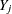with responses 1, …, g and a set of effects from its preceding variables, if the covariates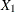,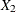, …,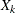associated with these effects within each group are approximately multivariate normal and the within-group covariance matrices are approximately equal, the discriminant function method (Brand, 1999, pp. 95–96) can be used to impute missing values for the variable.

Denote the group-specific means for covariates,, …,by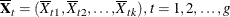then the pooled covariance matrix is computed as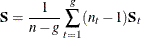where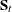is the within-group covariance matrix,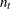is the group-specific sample size, and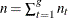is the total sample size.

In each imputation, new parameters of the group-specific means (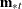), pooled covariance matrix (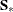), and prior probabilities of group membership (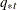) can be drawn from their corresponding posterior distributions (Schafer, 1997, p. 356).

#### Pooled Covariance Matrix and Group-Specific Means

For each imputation, the MI procedure uses either the fixed observed pooled covariance matrix (PCOV=FIXED) or a drawn pooled covariance matrix (PCOV=POSTERIOR) from its posterior distribution with a noninformative prior. That is,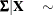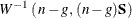where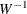is an inverted Wishart distribution.

The group-specific means are then drawn from their posterior distributions with a noninformative prior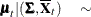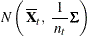See the section Bayesian Estimation of the Mean Vector and Covariance Matrix for a complete description of the inverted Wishart distribution and posterior distributions that use a noninformative prior.

#### Prior Probabilities of Group Membership

The prior probabilities are computed through the drawing of new group sample sizes. When the total sample size n is considered fixed, the group sample sizes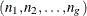have a multinomial distribution. New multinomial parameters (group sample sizes) can be drawn from their posterior distribution by using a Dirichlet prior with parameters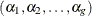.

After the new sample sizes are drawn from the posterior distribution of, the prior probabilitiesare computed proportionally to the drawn sample sizes.

See Schafer (1997, pp. 247–255) for a complete description of the Dirichlet prior.

#### Imputation Steps

The discriminant function method uses the following steps in each imputation to impute values for a nominal classification variablewith g responses:

1. Draw a pooled covariance matrixfrom its posterior distribution if the PCOV=POSTERIOR option is used.

2. For each group, draw group meansfrom the observed group mean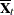and either the observed pooled covariance matrix (PCOV=FIXED) or the drawn pooled covariance matrix(PCOV=POSTERIOR).

3. For each group, compute or draw, prior probabilities of group membership, based on the PRIOR= option:

• PRIOR=EQUAL,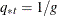, prior probabilities of group membership are all equal.

• PRIOR=PROPORTIONAL,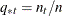, prior probabilities are proportional to their group sample sizes.

• PRIOR=JEFFREYS=, a noninformative Dirichlet prior with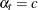is used.

• PRIOR=RIDGE=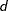, a ridge prior is used with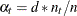for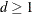and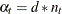for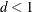.

4. With the group means, the pooled covariance matrix, and the prior probabilities of group membership, the discriminant function method derives linear discriminant function and computes the posterior probabilities of an observation belonging to each group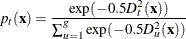where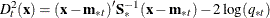is the generalized squared distance fromto group t.

5. Draw a random uniform variate u, between 0 and 1, for each observation with missing group value. With the posterior probabilities,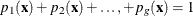, the discriminant function method imputes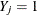if the value of u is less than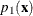,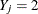if the value is greater than or equal tobut less than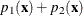, and so on.